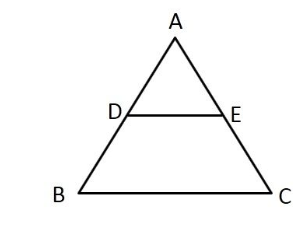# What is  basic proportional theorem and convers of basic proportional theorem?

Basic Proportionality Theorem:

If a line parallel to a side of a triangle intersects the remaining sides in two distinct points, then the line divides the side in the same proportion.Given: In ∆ABC, D and E are points on AB and AC respectively, such that DE is parallel to BC.

According to the basic proportionality theorem,

$\frac{AD}{BD} = \frac{AE}{EC}$.

Converse of Basic Proportionality Theorem: A line drawn to cut two sides of a triangle in equal proportion is parallel to the third side.

Given: In ∆ABC, D and E are mid-points on AB and AC respectively.

According to converse of the basic proportionality theorem,

$DE \parallel\ BC$